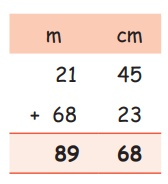Home | | Maths 4th Std | Addition of measures

## Chapter: 4th Maths : Term 1 Unit 4 : Measurements

EXAMPLE

Add 21m 45cm and 68m 23cm.step.1 Start from cm :(45+23)cm = 68cm

write 68 under the Centimetre column.

Step.2 Then add m : 21m + 68m = 89m

Write 89 under the metre column.

21m 45cm + 68m 23cm = 89m 68cm

EXAMPLE

Add 34m 91cm + 25m 42cmStep 1: Start from cm 91cm + 42cm = 133cm

In 133cm, write 33 under cm column and then add this 1cm to the metre column.

Step 2: Add 1m + 34m + 25m = 60m

34m 91cm + 25m 42cm = 60m 33cm

Tags : Measurements | Term 1 Chapter 4 | 4th Maths , 4th Maths : Term 1 Unit 4 : Measurements
Study Material, Lecturing Notes, Assignment, Reference, Wiki description explanation, brief detail
4th Maths : Term 1 Unit 4 : Measurements : Addition of measures | Measurements | Term 1 Chapter 4 | 4th Maths